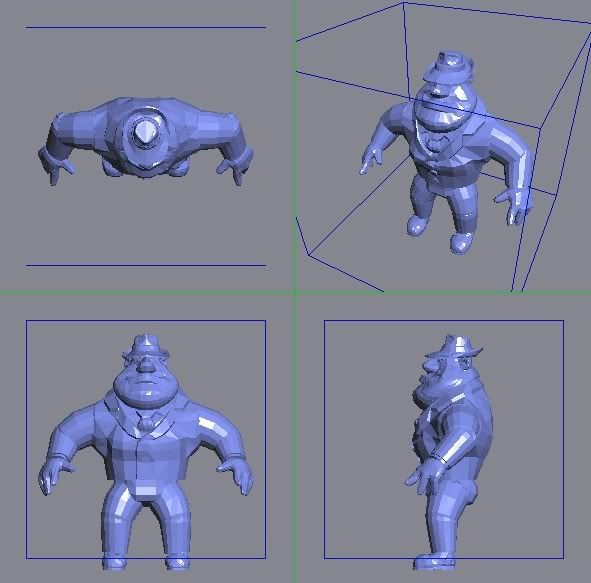# Problems with normalization code

My program can import Obj meshes from files and needs to (a) translate the model to the origin and (b) scale the model to a fit within a unit cube (0-1.0). My code mostly works except the model isn’t quite placed at the origin - its offset slightly and I can’t see what I’m doing wrongHere’s a pic of the problem - you can see the feet of the ‘Al’ model are outside the bounding box:mVertices is simply a STL <vector*> array of my vertex objects and ‘Vec3’ is simply my vector class with overloaded operators.

``````void Mesh::normalize()
{
using namespace std;
unsigned int i;
Vec3 oSize(0,0,0);

// calculate center point
for (i = 0; i < mVertices.size(); i++) {
oSize += mVertices.at(i)->getLocal();
}

Vec3 oCenter(0,0,0);

oCenter = (oSize / (mVertices.size()));

mCenterPoint.setLocal(oCenter);

Vec3 oVec3;
float fMaxDeviation = 0;

// calculate bounds
for (i = 0; i < mVertices.size(); i++)
{
oVec3 = mVertices.at(i)->getLocal();

// get max
fMaxDeviation = max(fMaxDeviation, oVec3.getX());
fMaxDeviation = max(fMaxDeviation, oVec3.getY());
fMaxDeviation = max(fMaxDeviation, oVec3.getZ());

// move to average position
mVertices.at(i)->setLocal(oVec3 - oCenter);
}

// normalize scale (0 - 1.0)
for (i = 0; i < mVertices.size(); i++)
{
oVec3 = mVertices.at(i)->getLocal();

mVertices.at(i)->setLocal(oVec3 /= fMaxDeviation);
}

}
``````

Any help appreciated!!!

First thing: You are calculating the fMaxDeviation from original vertex positions while you should calculate it from absolute value of “oVec3 - oCenter”. Otherwise the scale depends on position of the mesh before translation to the origin.

Second thing: You are calculating the center point as average of all positions however you should use center of bounding box instead.

Imagine that you have mesh with 99 points at position (1,1,1) and one point at position (0,0,0). For such mesh the averaged position would be (0.99, 0.99, 0.99) which is certainly not the ideal center.

Thanks for the reply Komat but I dont follow you - the bounding volume is already centered at the origin (0,0,0) so if I subtract from that instead of the vertex position it will have no affect (x-0). This I why I calculated the center from the average.

You have:
center = average(all)

You should have:
center = average(min, max)

``````vec3 minCorner = vertices;
vec3 maxCorner = vertices(0);

for (i = 1; i < vertices.size(); i++)
{
minCorner = min(minCorner, vertices[i]);
maxCorner = max(minCorner, vertices[i]);
}

vec3 center = (minCorner + maxCorner) / 2;
``````

min() and max() should work as described in GLSL specs: separately for each coordinate.

Originally posted by _new_horizon:
Thanks for the reply Komat but I dont follow you - the bounding volume is already centered at the origin (0,0,0)
The target bounding volume you wish to fit the object in is centered at the origin. The bounding box of the original geometry which I was talking about might be not. Otherwise there would be no need to translate the geometry to the origin because it would be already there.

The target bounding volume you wish to fit the object in is centered at the origin. The bounding box of the original geometry which I was talking about might be not. Otherwise there would be no need to translate the geometry to the origin because it would be already there.
But the imported mesh is just that - it has no bounding volume and to calculate that I need a center point and the maximum extents. That’s where the average comes in.

``:confused:``

Originally posted by _new_horizon:
But the imported mesh is just that - it has no bounding volume and to calculate that I need a center point and the maximum extents.

No. The bounding volume can be expressed in sveral ways depending on what is more handy for specific use case.

For bounding box bounding volume it can expressed as center and extents or it can be expressed as position of “minimum corner” and “maximum corner” of the bounding box (see post from k_szczech).

You can easily convert between the min&max representation which is easily calculated and the center&extends representation which is usefull for normalization. So you can simply calculate the min&max variant and convert it to the center&extends representation.